# Mean State

Period Mean (original grids) [K]
Model Period Mean (intersection) [K]
Model Period Mean (complement) [K]
Benchmark Period Mean (intersection) [K]
Benchmark Period Mean (complement) [K]
Bias [K]
RMSE [K]
Phase Shift [months]
Bias Score 
RMSE Score 
Seasonal Cycle Score 
Spatial Distribution Score 
Interannual Variability Score 
Overall Score 
Benchmark [-] 299.
CLM4 [-] 298. 298. 301. 298. 300. -0.366 1.05 0.353 0.460 0.755 0.960 0.942 0.927 0.800
CLM4.5 [-] 298. 298. 300. 298. 300. -0.147 0.947 0.322 0.502 0.779 0.967 0.958 0.944 0.821
CLM5 [-] 299. 299. 301. 298. 300. 0.167 0.738 0.323 0.625 0.793 0.963 0.985 0.956 0.853
Period Mean (original grids) [K]
Model Period Mean (intersection) [K]
Model Period Mean (complement) [K]
Benchmark Period Mean (intersection) [K]
Benchmark Period Mean (complement) [K]
Bias [K]
RMSE [K]
Phase Shift [months]
Bias Score 
RMSE Score 
Seasonal Cycle Score 
Spatial Distribution Score 
Interannual Variability Score 
Overall Score 
Benchmark [-] 300.
CLM4 [-] 300. 300. 301. 300. 300. 0.0789 0.911 0.228 0.757 0.790 0.983 1.00 0.946 0.878
CLM4.5 [-] 300. 301. 301. 300. 300. 0.316 0.883 0.221 0.743 0.824 0.983 0.997 0.962 0.889
CLM5 [-] 301. 301. 301. 300. 300. 0.521 0.852 0.195 0.730 0.854 0.985 0.996 0.969 0.898
Period Mean (original grids) [K]
Model Period Mean (intersection) [K]
Model Period Mean (complement) [K]
Benchmark Period Mean (intersection) [K]
Benchmark Period Mean (complement) [K]
Bias [K]
RMSE [K]
Phase Shift [months]
Bias Score 
RMSE Score 
Seasonal Cycle Score 
Spatial Distribution Score 
Interannual Variability Score 
Overall Score 
Benchmark [-] 295.
CLM4 [-] 295. 295. 296. 295. 297. 0.0992 0.933 0.410 0.687 0.827 0.948 1.00 0.918 0.868
CLM4.5 [-] 295. 295. 296. 295. 297. 0.336 0.850 0.334 0.718 0.853 0.960 0.999 0.929 0.885
CLM5 [-] 296. 295. 297. 295. 297. 0.445 0.865 0.404 0.708 0.867 0.945 1.00 0.948 0.889
Period Mean (original grids) [K]
Model Period Mean (intersection) [K]
Model Period Mean (complement) [K]
Benchmark Period Mean (intersection) [K]
Benchmark Period Mean (complement) [K]
Bias [K]
RMSE [K]
Phase Shift [months]
Bias Score 
RMSE Score 
Seasonal Cycle Score 
Spatial Distribution Score 
Interannual Variability Score 
Overall Score 
Benchmark [-] 262.
CLM4 [-] 258. 258. 262. 262. 266. -2.90 3.47 0.0203 0.830 0.894 0.999 0.996 0.916 0.921
CLM4.5 [-] 259. 259. 262. 262. 266. -2.58 3.20 0.0161 0.846 0.895 0.999 0.996 0.912 0.924
CLM5 [-] 260. 260. 263. 262. 266. -1.00 1.60 0.0159 0.921 0.943 0.999 0.999 0.921 0.954
Period Mean (original grids) [K]
Model Period Mean (intersection) [K]
Model Period Mean (complement) [K]
Benchmark Period Mean (intersection) [K]
Benchmark Period Mean (complement) [K]
Bias [K]
RMSE [K]
Phase Shift [months]
Bias Score 
RMSE Score 
Seasonal Cycle Score 
Spatial Distribution Score 
Interannual Variability Score 
Overall Score 
Benchmark [-] 299.
CLM4 [-] 298. 298. 299. 299. 299. -0.555 1.01 0.773 0.330 0.604 0.890 0.973 0.850 0.708
CLM4.5 [-] 299. 298. 299. 299. 299. -0.452 0.944 0.717 0.354 0.620 0.899 0.978 0.864 0.723
CLM5 [-] 299. 299. 300. 299. 299. -0.0330 0.793 0.616 0.415 0.681 0.912 0.996 0.880 0.761
Period Mean (original grids) [K]
Model Period Mean (intersection) [K]
Model Period Mean (complement) [K]
Benchmark Period Mean (intersection) [K]
Benchmark Period Mean (complement) [K]
Bias [K]
RMSE [K]
Phase Shift [months]
Bias Score 
RMSE Score 
Seasonal Cycle Score 
Spatial Distribution Score 
Interannual Variability Score 
Overall Score 
Benchmark [-] 271.
CLM4 [-] 269. 269. 273. 271. 274. -2.43 2.85 0.00954 0.839 0.906 0.999 0.997 0.969 0.936
CLM4.5 [-] 269. 269. 273. 271. 274. -2.28 2.73 0.00954 0.847 0.906 0.999 0.998 0.971 0.938
CLM5 [-] 271. 270. 274. 271. 274. -0.737 1.06 0.00890 0.938 0.961 0.999 0.999 0.981 0.973
Period Mean (original grids) [K]
Model Period Mean (intersection) [K]
Model Period Mean (complement) [K]
Benchmark Period Mean (intersection) [K]
Benchmark Period Mean (complement) [K]
Bias [K]
RMSE [K]
Phase Shift [months]
Bias Score 
RMSE Score 
Seasonal Cycle Score 
Spatial Distribution Score 
Interannual Variability Score 
Overall Score 
Benchmark [-] 280.
CLM4 [-] 279. 279. 287. 280. 285. -0.945 1.80 0.0264 0.862 0.913 0.998 1.00 0.938 0.937
CLM4.5 [-] 280. 279. 287. 280. 285. -0.792 1.70 0.0264 0.870 0.915 0.998 1.00 0.942 0.940
CLM5 [-] 280. 280. 287. 280. 285. -0.0730 1.17 0.0271 0.900 0.948 0.998 1.00 0.963 0.960
Period Mean (original grids) [K]
Model Period Mean (intersection) [K]
Model Period Mean (complement) [K]
Benchmark Period Mean (intersection) [K]
Benchmark Period Mean (complement) [K]
Bias [K]
RMSE [K]
Phase Shift [months]
Bias Score 
RMSE Score 
Seasonal Cycle Score 
Spatial Distribution Score 
Interannual Variability Score 
Overall Score 
Benchmark [-] 297.
CLM4 [-] 296. 296. 300. 297. 300. -0.323 1.31 0.180 0.742 0.847 0.982 0.988 0.909 0.886
CLM4.5 [-] 297. 297. 300. 297. 300. -0.121 1.24 0.181 0.747 0.855 0.982 0.993 0.919 0.892
CLM5 [-] 297. 297. 300. 297. 300. 0.195 1.12 0.170 0.749 0.886 0.983 0.998 0.940 0.907
Period Mean (original grids) [K]
Model Period Mean (intersection) [K]
Model Period Mean (complement) [K]
Benchmark Period Mean (intersection) [K]
Benchmark Period Mean (complement) [K]
Bias [K]
RMSE [K]
Phase Shift [months]
Bias Score 
RMSE Score 
Seasonal Cycle Score 
Spatial Distribution Score 
Interannual Variability Score 
Overall Score 
Benchmark [-] 264.
CLM4 [-] 261. 261. 262. 264. 264. -3.09 3.70 0.00943 0.816 0.877 0.999 0.996 0.960 0.921
CLM4.5 [-] 261. 261. 262. 264. 264. -2.91 3.46 0.0496 0.825 0.886 0.997 0.997 0.960 0.925
CLM5 [-] 263. 263. 264. 264. 264. -1.09 1.43 0.0193 0.929 0.948 0.999 0.999 0.970 0.965
Period Mean (original grids) [K]
Model Period Mean (intersection) [K]
Model Period Mean (complement) [K]
Benchmark Period Mean (intersection) [K]
Benchmark Period Mean (complement) [K]
Bias [K]
RMSE [K]
Phase Shift [months]
Bias Score 
RMSE Score 
Seasonal Cycle Score 
Spatial Distribution Score 
Interannual Variability Score 
Overall Score 
Benchmark [-] 295.
CLM4 [-] 295. 295. 293. 295. 294. 0.0117 0.641 0.110 0.898 0.914 0.992 0.997 0.935 0.942
CLM4.5 [-] 295. 295. 293. 295. 294. 0.00772 0.650 0.111 0.897 0.913 0.992 0.997 0.931 0.941
CLM5 [-] 295. 295. 293. 295. 294. 0.323 0.624 0.0892 0.890 0.929 0.994 0.999 0.950 0.949
Period Mean (original grids) [K]
Model Period Mean (intersection) [K]
Model Period Mean (complement) [K]
Benchmark Period Mean (intersection) [K]
Benchmark Period Mean (complement) [K]
Bias [K]
RMSE [K]
Phase Shift [months]
Bias Score 
RMSE Score 
Seasonal Cycle Score 
Spatial Distribution Score 
Interannual Variability Score 
Overall Score 
Benchmark [-] 282.
CLM4 [-] 281. 281. 283. 282. 286. -1.00 1.69 0.124 0.836 0.870 0.992 0.980 0.906 0.909
CLM4.5 [-] 281. 281. 283. 282. 286. -1.02 1.67 0.128 0.834 0.874 0.991 0.985 0.908 0.911
CLM5 [-] 281. 281. 284. 282. 286. -0.172 0.983 0.147 0.893 0.924 0.990 0.997 0.946 0.946
Period Mean (original grids) [K]
Model Period Mean (intersection) [K]
Model Period Mean (complement) [K]
Benchmark Period Mean (intersection) [K]
Benchmark Period Mean (complement) [K]
Bias [K]
RMSE [K]
Phase Shift [months]
Bias Score 
RMSE Score 
Seasonal Cycle Score 
Spatial Distribution Score 
Interannual Variability Score 
Overall Score 
Benchmark [-] 287.
CLM4 [-] 281. 286. 243. 287. 292. -0.921 1.65 0.173 0.770 0.859 0.981 0.993 0.931 0.899
CLM4.5 [-] 281. 286. 243. 287. 292. -0.792 1.58 0.164 0.782 0.865 0.983 0.993 0.936 0.904
CLM5 [-] 282. 286. 244. 287. 292. -0.107 1.02 0.157 0.821 0.900 0.982 0.998 0.952 0.926
Period Mean (original grids) [K]
Model Period Mean (intersection) [K]
Model Period Mean (complement) [K]
Benchmark Period Mean (intersection) [K]
Benchmark Period Mean (complement) [K]
Bias [K]
RMSE [K]
Phase Shift [months]
Bias Score 
RMSE Score 
Seasonal Cycle Score 
Spatial Distribution Score 
Interannual Variability Score 
Overall Score 
Benchmark [-] 296.
CLM4 [-] 296. 296. 296. 296. 297. -0.125 1.01 0.152 0.882 0.921 0.989 1.00 0.958 0.945
CLM4.5 [-] 296. 296. 296. 296. 297. -0.0557 0.978 0.149 0.886 0.923 0.989 1.00 0.957 0.946
CLM5 [-] 296. 296. 297. 296. 297. 0.240 0.867 0.138 0.891 0.939 0.990 1.00 0.965 0.954
Period Mean (original grids) [K]
Model Period Mean (intersection) [K]
Model Period Mean (complement) [K]
Benchmark Period Mean (intersection) [K]
Benchmark Period Mean (complement) [K]
Bias [K]
RMSE [K]
Phase Shift [months]
Bias Score 
RMSE Score 
Seasonal Cycle Score 
Spatial Distribution Score 
Interannual Variability Score 
Overall Score 
Benchmark [-] 265.
CLM4 [-] 262. 262. 260. 265. 266. -2.77 3.36 0.0230 0.809 0.871 0.998 0.994 0.942 0.914
CLM4.5 [-] 262. 262. 260. 265. 266. -2.60 3.21 0.0179 0.819 0.872 0.999 0.994 0.938 0.916
CLM5 [-] 264. 264. 261. 265. 266. -0.989 1.43 0.0106 0.920 0.935 0.999 0.998 0.945 0.955
Period Mean (original grids) [K]
Model Period Mean (intersection) [K]
Model Period Mean (complement) [K]
Benchmark Period Mean (intersection) [K]
Benchmark Period Mean (complement) [K]
Bias [K]
RMSE [K]
Phase Shift [months]
Bias Score 
RMSE Score 
Seasonal Cycle Score 
Spatial Distribution Score 
Interannual Variability Score 
Overall Score 
Benchmark [-] 296.
CLM4 [-] 295. 295. 298. 296. 298. -0.0641 1.27 0.269 0.664 0.825 0.977 0.996 0.912 0.867
CLM4.5 [-] 295. 295. 298. 296. 298. -0.107 1.25 0.293 0.671 0.813 0.974 1.00 0.908 0.863
CLM5 [-] 295. 295. 298. 296. 298. 0.206 1.20 0.263 0.668 0.847 0.977 1.00 0.925 0.877
Period Mean (original grids) [K]
Model Period Mean (intersection) [K]
Model Period Mean (complement) [K]
Benchmark Period Mean (intersection) [K]
Benchmark Period Mean (complement) [K]
Bias [K]
RMSE [K]
Phase Shift [months]
Bias Score 
RMSE Score 
Seasonal Cycle Score 
Spatial Distribution Score 
Interannual Variability Score 
Overall Score 
Benchmark [-] 286.
CLM4 [-] 285. 285. 293. 286. 292. -1.07 1.41 0.0428 0.880 0.914 0.997 0.997 0.952 0.942
CLM4.5 [-] 285. 285. 293. 286. 292. -0.971 1.35 0.0433 0.890 0.913 0.997 0.997 0.955 0.944
CLM5 [-] 286. 286. 294. 286. 292. -0.0907 0.670 0.0345 0.945 0.947 0.998 0.999 0.968 0.967
Period Mean (original grids) [K]
Model Period Mean (intersection) [K]
Model Period Mean (complement) [K]
Benchmark Period Mean (intersection) [K]
Benchmark Period Mean (complement) [K]
Bias [K]
RMSE [K]
Phase Shift [months]
Bias Score 
RMSE Score 
Seasonal Cycle Score 
Spatial Distribution Score 
Interannual Variability Score 
Overall Score 
Benchmark [-] 275.
CLM4 [-] 272. 272. 276. 274. 278. -2.21 2.59 0.0546 0.814 0.895 0.996 0.974 0.964 0.923
CLM4.5 [-] 272. 272. 276. 274. 278. -2.08 2.48 0.0553 0.824 0.895 0.996 0.975 0.965 0.925
CLM5 [-] 274. 274. 277. 274. 278. -0.630 1.01 0.0424 0.923 0.947 0.997 0.995 0.971 0.964
Period Mean (original grids) [K]
Model Period Mean (intersection) [K]
Model Period Mean (complement) [K]
Benchmark Period Mean (intersection) [K]
Benchmark Period Mean (complement) [K]
Bias [K]
RMSE [K]
Phase Shift [months]
Bias Score 
RMSE Score 
Seasonal Cycle Score 
Spatial Distribution Score 
Interannual Variability Score 
Overall Score 
Benchmark [-] 284.
CLM4 [-] 282. 283. 286. 284. 286. -0.886 1.75 0.0307 0.846 0.887 0.998 0.994 0.928 0.923
CLM4.5 [-] 282. 283. 286. 284. 286. -0.805 1.75 0.0295 0.851 0.879 0.998 0.996 0.932 0.922
CLM5 [-] 283. 283. 287. 284. 286. -0.0645 1.18 0.0249 0.883 0.924 0.998 1.00 0.965 0.949
Period Mean (original grids) [K]
Model Period Mean (intersection) [K]
Model Period Mean (complement) [K]
Benchmark Period Mean (intersection) [K]
Benchmark Period Mean (complement) [K]
Bias [K]
RMSE [K]
Phase Shift [months]
Bias Score 
RMSE Score 
Seasonal Cycle Score 
Spatial Distribution Score 
Interannual Variability Score 
Overall Score 
Benchmark [-] 299.
CLM4 [-] 298. 298. 300. 299. 299. -0.539 0.965 0.523 0.534 0.723 0.925 0.979 0.901 0.797
CLM4.5 [-] 299. 299. 300. 299. 299. -0.364 0.863 0.491 0.596 0.732 0.932 0.982 0.911 0.814
CLM5 [-] 299. 299. 300. 299. 299. -0.0597 0.717 0.460 0.654 0.763 0.936 0.996 0.939 0.842
Period Mean (original grids) [K]
Model Period Mean (intersection) [K]
Model Period Mean (complement) [K]
Benchmark Period Mean (intersection) [K]
Benchmark Period Mean (complement) [K]
Bias [K]
RMSE [K]
Phase Shift [months]
Bias Score 
RMSE Score 
Seasonal Cycle Score 
Spatial Distribution Score 
Interannual Variability Score 
Overall Score 
Benchmark [-] 296.
CLM4 [-] 296. 296. 296. 296. 293. -0.282 0.892 0.258 0.740 0.823 0.968 0.992 0.902 0.875
CLM4.5 [-] 296. 296. 296. 296. 293. -0.108 0.818 0.271 0.758 0.831 0.968 0.990 0.914 0.882
CLM5 [-] 296. 296. 296. 296. 293. 0.242 0.663 0.248 0.776 0.866 0.969 0.998 0.941 0.903
Period Mean (original grids) [K]
Model Period Mean (intersection) [K]
Model Period Mean (complement) [K]
Benchmark Period Mean (intersection) [K]
Benchmark Period Mean (complement) [K]
Bias [K]
RMSE [K]
Phase Shift [months]
Bias Score 
RMSE Score 
Seasonal Cycle Score 
Spatial Distribution Score 
Interannual Variability Score 
Overall Score 
Benchmark [-] 284.
CLM4 [-] 283. 283. 287. 284. 285. -0.572 1.82 0.225 0.641 0.810 0.973 0.995 0.927 0.860
CLM4.5 [-] 284. 283. 287. 284. 285. -0.321 1.73 0.219 0.655 0.815 0.974 0.997 0.928 0.864
CLM5 [-] 284. 284. 288. 284. 285. 0.0843 1.53 0.206 0.670 0.855 0.975 0.999 0.942 0.883

# Temporally integrated period mean

BENCHMARK MEAN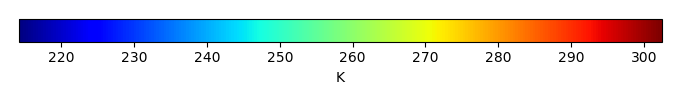MODEL MEANBIAS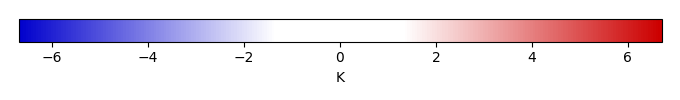BIAS SCORERMSE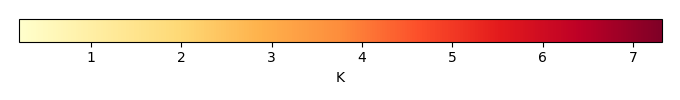RMSE SCOREBENCHMARK INTERANNUAL VARIABILITY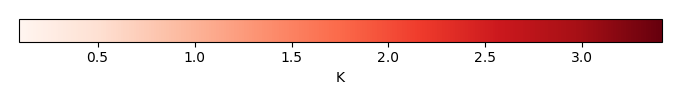MODEL INTERANNUAL VARIABILITYINTERANNUAL VARIABILITY SCOREBENCHMARK MAX MONTHMODEL MAX MONTHDIFFERENCE IN MAX MONTH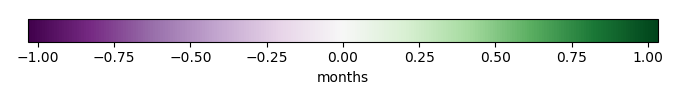SEASONAL CYCLE SCORESPATIAL TAYLOR DIAGRAMMODEL COLORS# Spatially integrated regional mean

MODEL COLORSREGIONAL MEANANNUAL CYCLEMONTHLY ANOMALYANNUAL CYCLE# All Models

BenchmarkCLM4CLM4.5CLM5# Data Information

creation_date: Fri Nov 1 11:52:30 PDT 2013

source_file: This product is generated from monthly CRU TS3.21 Mean Temperature observations

title: derived CRU high resolution (0.5x0.5) Mean Temperature

Approach: I simply read the monthly surface air temperature from original file, and separated the time series by a month and saved them by each month and each year.

Temporal resolution: monthly

General information: This product was derived from CRU v3.21 product.

Spatial resolution: 0.5x0.5 degree